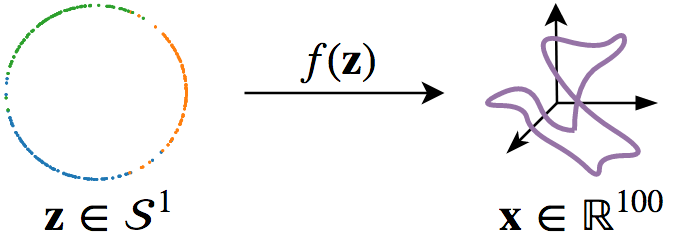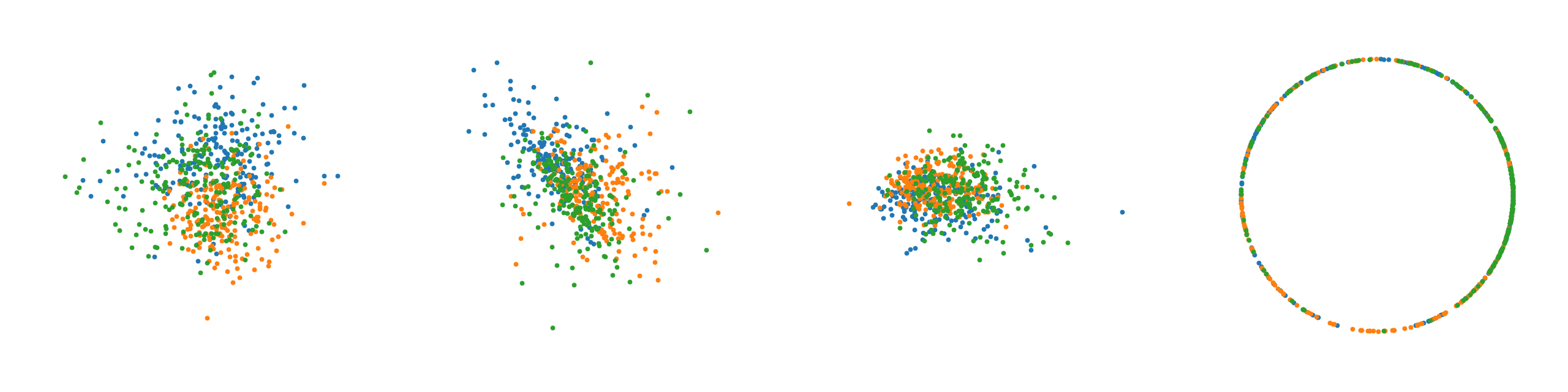# What is a $\mathcal{S}$-VAE?

A $\mathcal{S}$-VAE is a variational auto-encoder with a hyperspherical latent space. In our paper we propose to use the von Mises-Fisher (vMF) distribution to achieve this, under which the Uniform distribution on the hypersphere is recovered as a special case. Hence this approach allows for a truly uninformative prior, with a clear advantage for data with a hyperspherical latent representation.

## Why use a $\mathcal{S}$-VAE instead of a $\mathcal{N}$-VAE?

VAEs using the Gaussian variational prior/posterior ($\mathcal{N}$-VAE) are the default choice. But although the Gaussian is mathematically convenient, it exhibits some problematic properties both in low and high dimenions; in low dimensions origin gravity takes place, where points are drawn to the origin. In high dimensions, the so called ‘soap-bubble-effect’ is observed, where the vast majority of the probability mass is concentrated on the hyperspherical shell.

Additionally, on a more fundamental level there is the problem of manifold mapping: if the data to be modeled, lies on a latent manifold not homeomorphic to $\mathbb{R}^M$, information is lost. We will show an example of this last phenomenon next.

## Example: Recovering a Hyperspherical Latent Structuredata: We first generate samples from a mixture of three vMFs on the circle, $\mathcal{S}^1$, which subsequently are mapped into the higher dimensional $\mathbb{R}^{100}$ by applying a noisy, non-linear transformation.

models: After this, we in turn train an auto-encoder, a $\mathcal{N}$-VAE, and a $\mathcal{S}$-VAE. We further investigate the behavior of the $\mathcal{N}$-VAE, by training a model using a scaled down KL divergence.From left to right, plots of learned latent space representations of a. the Auto-Encoder, b. the $\mathcal{N}$-VAE, c. the $\mathcal{N}$-VAE with a scaled down KL divergence, and d. the $\mathcal{S}$-VAE.

Note that as expected the auto-encoder mostly recovers the original circular latent space as there are no distributional restrictions. We clearly observe for the $\mathcal{N}$-VAE that points collapse around the origin due to the KL, which is much less pronounced when the KL contribution is scaled down. Lastly, the $\mathcal{S}$-VAE almost perfectly recovers the original circular latent space. The observed behavior confirms our intuition.

## Citation

Davidson, T. R., Falorsi, L., De Cao, N., Kipf, T., and Tomczak,
J. M. (2018). Hyperspherical Variational Auto-Encoders. 34th
Conference on Uncertainty in Artificial Intelligence (UAI-18).


BibTeX format:

@article{s-vae18,
title={Hyperspherical Variational Auto-Encoders},
author={Davidson, Tim R. and
Falorsi, Luca and
De Cao, Nicola and
Kipf, Thomas and
Tomczak, Jakub M.},
journal={34th Conference on Uncertainty in Artificial Intelligence (UAI-18)},
year={2018}
}